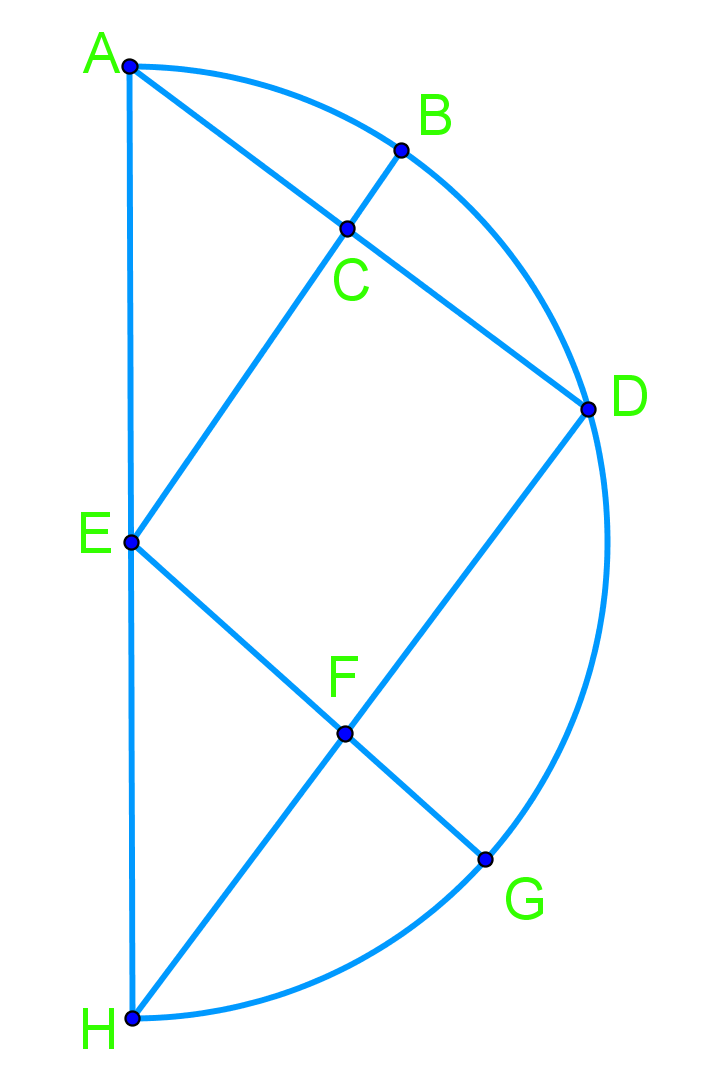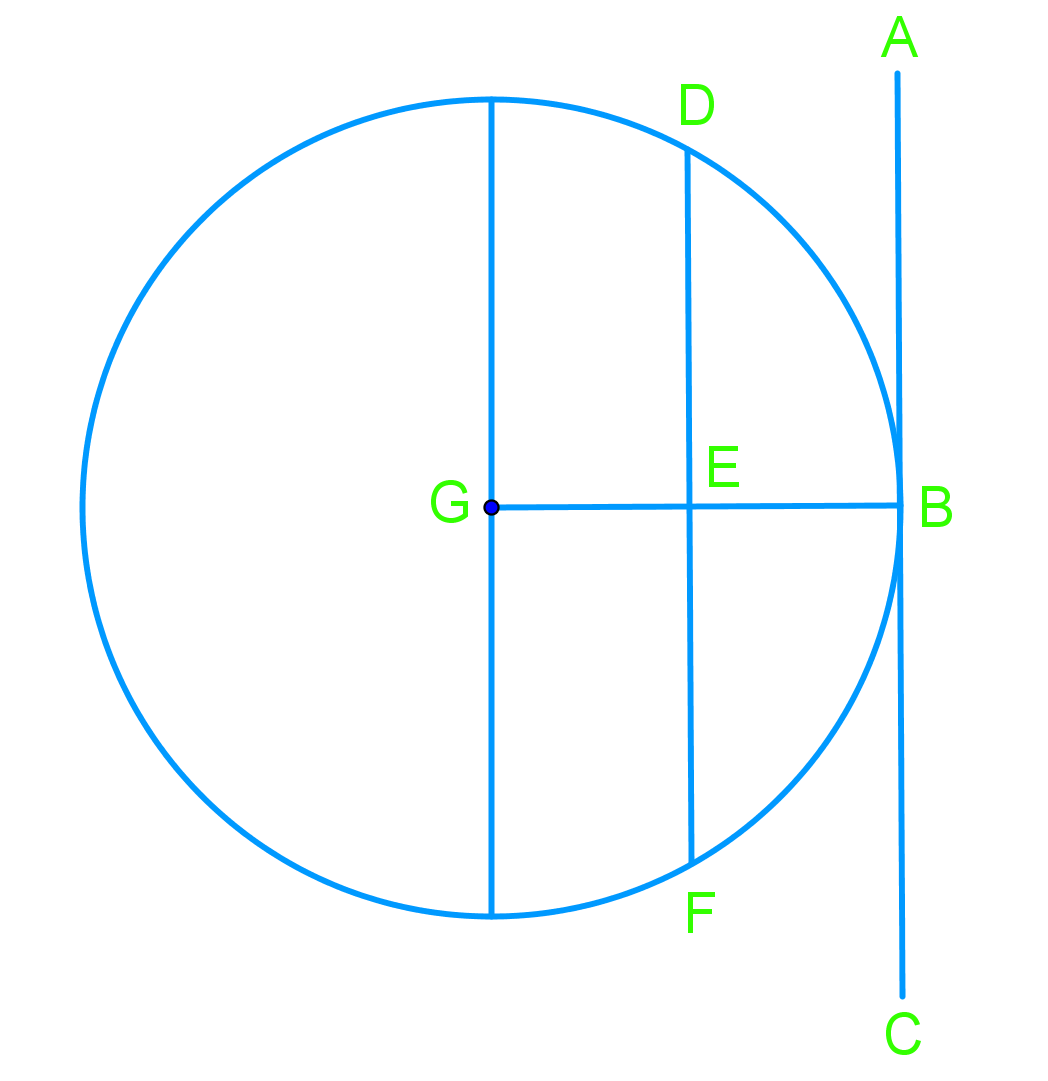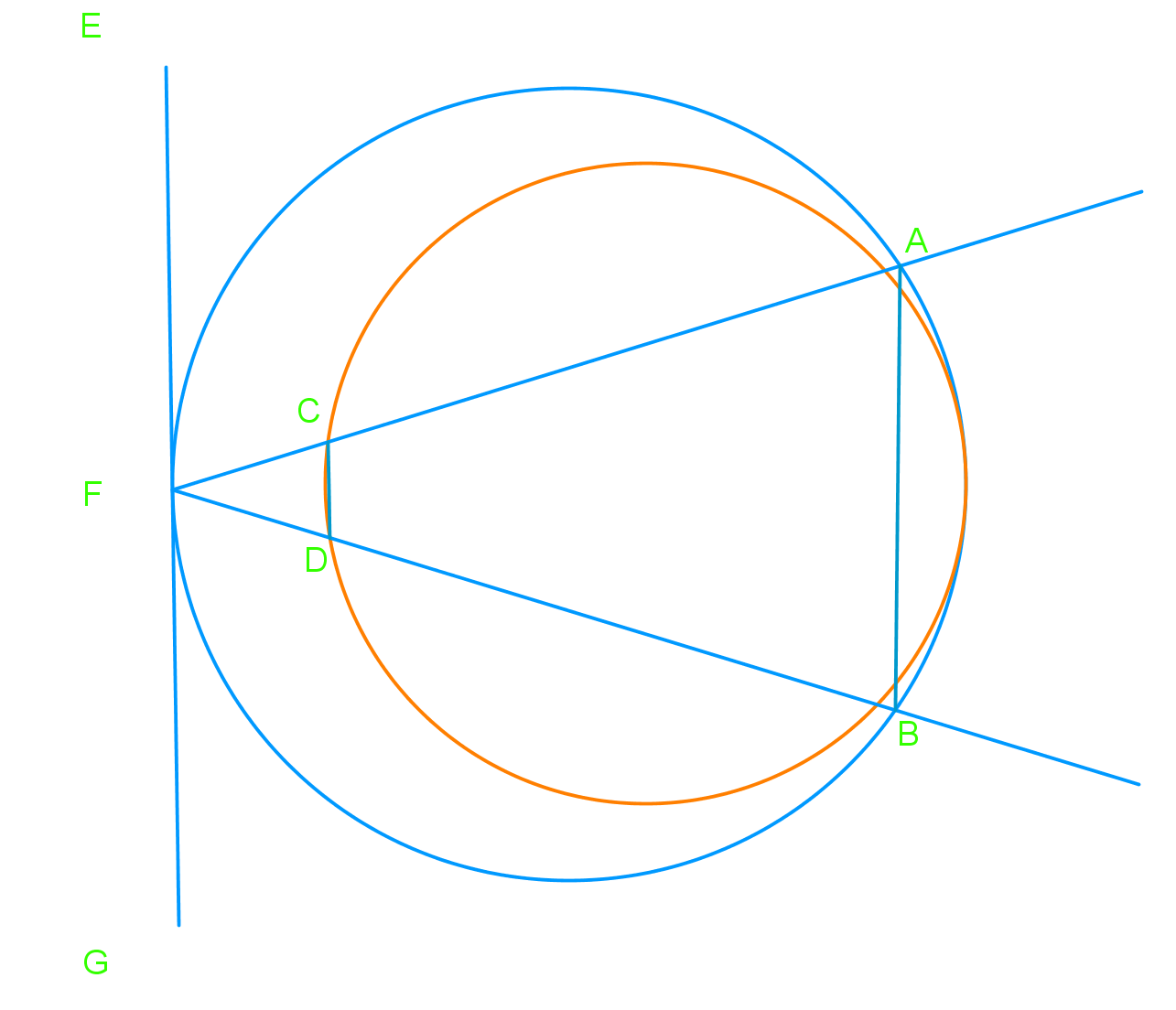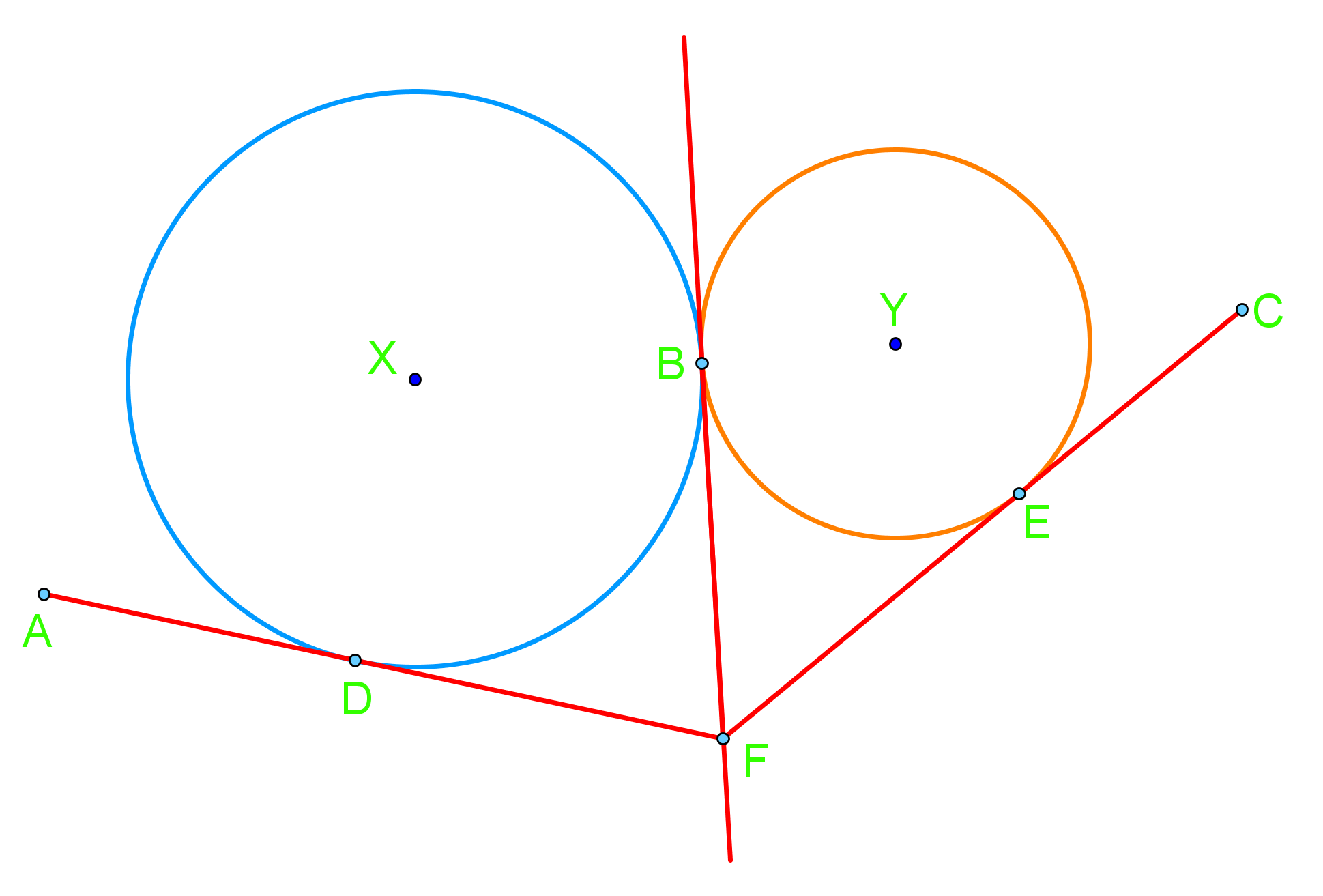# Circle chord, tangent, and inscribed angles proofs#### Everything You Need in One Place

Homework problems? Exam preparation? Trying to grasp a concept or just brushing up the basics? Our extensive help & practice library have got you covered.#### Learn and Practice With Ease

Our proven video lessons ease you through problems quickly, and you get tonnes of friendly practice on questions that trip students up on tests and finals.#### Instant and Unlimited Help

Our personalized learning platform enables you to instantly find the exact walkthrough to your specific type of question. Activate unlimited help now!##### Intros
###### Lessons
1. What is a chord?
2. What is a tangent?
##### Examples
###### Lessons
1. Given: 2AC =AD, 2DF=DH Prove: $\angle$BEG = 90°1. Given: AC is tangent at B, AC is parallel to DF Prove: arc BD = arc BF Given: 2AC =AD, 2DF=DH Prove: $\angle$BEG = 90°1. Given: EG is tangent at F, AB is parallel to CD Prove: $\angle$BAF = $\angle$DCF Given: 2AC =AD, 2DF=DH Prove: $\angle$BEG = 90°1. Given: AF is tangent to circle X at D, CF is tangent to circle Y at E. DF tangent to both circles Prove: DF = EF Given: 2AC =AD, 2DF=DH Prove: $\angle$BEG = 90°